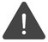# FP_TO_NUM

Convert from floating-point to implied-decimal

 WTSupported in traditional Synergy on Windows USupported on UNIX VSupported on OpenVMS
```xcall FP_TO_NUM(float, number)
```

Arguments

float

A variable that contains a native floating-point number (C “double” data type) to convert to an implied-decimal number. (a8)

number

Returned with an implied-decimal number or alpha string. (a23 or n)

Discussion

The FP_TO_NUM subroutine converts a floating-point number to an implied-decimal number.

If the second arguments to the FP_TO_NUM subroutine is alpha, the returned format is as follows:

X.YYYYYYYYYYYYYYYY eNNN

Therefore, you can inspect all values of floating-point numbers. For very large and very small floating-point values, the implied-decimal value is returned as zero.To avoid receiving invalid results when using the floating-point routines, do not use the %IMPLIED function with this call. %IMPLIED performs a transformation of the data and allocates temporary memory for the result. Therefore, using %IMPLIED causes temporary memory, rather than the memory location passed to %IMPLIED, to be modified.

Examples

See Sample program.